Courses

# Worksheet - Be My Multiply Notes | EduRev

## Class 5 : Worksheet - Be My Multiply Notes | EduRev

The document Worksheet - Be My Multiply Notes | EduRev is a part of the Class 5 Course Mathematics for Class 5: NCERT.
All you need of Class 5 at this link: Class 5

Q.1. Find the product.
(a)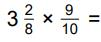____
Ans.
2(37/40)

(b)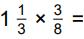____
Ans.
1/2

(c)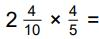____
Ans.
1(23/25)

(d)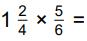____
Ans.
1(1/4)

(e)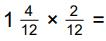____
Ans.
2/9

(f)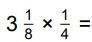____
Ans.
25/32

(g)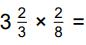____
Ans.
11/12

(h)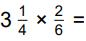____
Ans.
1(1/12)

(i)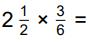____
Ans.
1(1/4)

(j)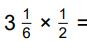____
Ans.
1(7/12)

(k)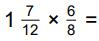____
Ans.
1(3/16)

(l)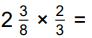____
Ans.
1(7/12)

(m)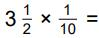____
Ans.
7/20

(n)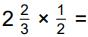____
Ans.
1(1/3)

(o)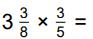____
Ans.
2(1/40)

(p)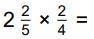____
Ans.
1(1/5)

(q)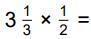____
Ans.
1(2/3)

(r)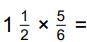____
Ans.
1(1/4)

(s)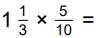____
Ans.
2/3

(t)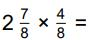____
Ans.
1(7/16)

Q.2. Practice the questions given in the worksheet on factors and multiples.
(a) Find out the even numbers.
27, 36, 48, 125, 360, 453, 518, 423, 54, 58, 917, 186, 423, 928, 358
Ans.
36, 48, 360, 518, 54, 58, 186, 928, 358

(b) Find out the odd numbers.
10, 45, 78, 146, 347, 543, 495, 638, 497, 968, 729, 427, 624, 572
Ans.
45, 347, 543, 495, 497, 729, 427

(c) Write the factors of the following:
(i) 27
(ii) 32
(iii) 18
(iv) 45
(v) 25
(vi) 56
(vii) 68
(viii) 38
(ix) 72
(x) 56
Ans.

(i) 1, 3, 9, 27
(ii) 1, 2, 4, 8, 16, 32
(iii) 1, 2, 3, 6, 9, 18
(iv) 1, 3, 5, 9, 15, 45
(v) 1, 5, 25
(vi) 1, 2, 4, 7, 8, 14, 28, 56
(vii) 1, 2, 4, 17, 34, 68
(viii) 1, 2, 19, 38
(ix) 1, 2, 3, 4, 6, 8, 9, 12, 18, 24, 36, 72
(x) 1, 2, 4, 7, 8, 14, 28, 56

(d) Write the first five multiples of the following:
(i) 4
(ii) 3
(iii) 7
(iv) 9
(v) 5
(vi) 8
(vii) 12
(viii) 15
Ans.

(i) 4, 8, 12, 16, 20
(ii) 3, 6, 9, 12, 15
(iii) 7, 14, 21, 28, 35
(iv) 9, 18, 27, 36, 45
(v) 5, 10, 15, 20, 25
(vi) 8, 16, 24, 32, 40
(vii) 12, 24, 36, 48, 60
(viii) 15, 30, 45, 60, 75

(e) Find the first three multiples of 8.
Ans.
8, 16, 24

(f) Find the missing factors.
(i) 7 × ___ = 56
(ii) 5 × ___ = 30
(iii) ___ × 3 = 24
(iv) ___ × 9 = 72
(v) 6 × ___ = 48
(vi) 8 × ___ = 72
Ans.

(i) 8
(ii) 6
(iii) 8
(iv) 8
(v) 8
(vi) 9

(g) Write the multiples of 6 which are greater than 20 and less than 50.
Ans.
24, 30, 36, 42, 48

(h) Write all the prime numbers between 1 and 15.
Ans.
2, 3, 5, 7, 11, 13

(i) Write all the composite numbers between 1 and 30.
Ans.
4, 6, 8, 9, 10, 12, 14, 15, 16, 18, 20, 21, 22, 24, 25, 26, 27, 28, 30

(j) Write all the prime numbers between the following:
(i) 31 and 50
(ii) 50 and 90
(iii) 61 and 80
(iv) 91 and 100
Ans.

(i) 31, 37, 41, 43, 47
(ii) 53, 59
(iii) 61, 67, 71, 73, 79
(iv) 97

Q.3. Find the missing numbers:
(a) ____ × 98 = 98000
Ans.
1000

(b) 763 × ____ = 7630
Ans.
10

(c) ____  × 807 = 8070
Ans.
10

(d) 1000 × ____ = 663000
Ans.
663

(e) 686 × ____ = 686000
Ans.
1000

(f) 1000 × ____ = 715000
Ans.
715

(g) ____ × 10 = 8900
Ans.
890

(h) ____ × 1000 = 483000
Ans.
483

(i) ____ × 1000 = 748000
Ans.
748

(j) ____ × 718 = 718000
Ans.
1000

Offer running on EduRev: Apply code STAYHOME200 to get INR 200 off on our premium plan EduRev Infinity!

## Mathematics for Class 5: NCERT

40 videos|43 docs|39 tests

,

,

,

,

,

,

,

,

,

,

,

,

,

,

,

,

,

,

,

,

,

;